Test: AC Power Analysis - 1

# Test: AC Power Analysis - 1

Test Description

## 10 Questions MCQ Test GATE Electrical Engineering (EE) 2023 Mock Test Series | Test: AC Power Analysis - 1

Test: AC Power Analysis - 1 for Electrical Engineering (EE) 2023 is part of GATE Electrical Engineering (EE) 2023 Mock Test Series preparation. The Test: AC Power Analysis - 1 questions and answers have been prepared according to the Electrical Engineering (EE) exam syllabus.The Test: AC Power Analysis - 1 MCQs are made for Electrical Engineering (EE) 2023 Exam. Find important definitions, questions, notes, meanings, examples, exercises, MCQs and online tests for Test: AC Power Analysis - 1 below.
Solutions of Test: AC Power Analysis - 1 questions in English are available as part of our GATE Electrical Engineering (EE) 2023 Mock Test Series for Electrical Engineering (EE) & Test: AC Power Analysis - 1 solutions in Hindi for GATE Electrical Engineering (EE) 2023 Mock Test Series course. Download more important topics, notes, lectures and mock test series for Electrical Engineering (EE) Exam by signing up for free. Attempt Test: AC Power Analysis - 1 | 10 questions in 30 minutes | Mock test for Electrical Engineering (EE) preparation | Free important questions MCQ to study GATE Electrical Engineering (EE) 2023 Mock Test Series for Electrical Engineering (EE) Exam | Download free PDF with solutions
 1 Crore+ students have signed up on EduRev. Have you?
Test: AC Power Analysis - 1 - Question 1

### The value of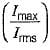for the waveform shown below is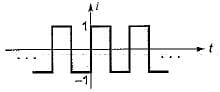Detailed Solution for Test: AC Power Analysis - 1 - Question 1

For the square wave shown in given figure,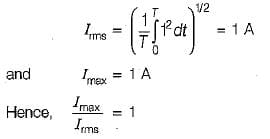Test: AC Power Analysis - 1 - Question 2

### For the idealized full wave rectifier system the average and rms value of the voltage is

Test: AC Power Analysis - 1 - Question 3

### The instantaneous power delivered to the 5 Ω resistor at t = 0 in the given circuit is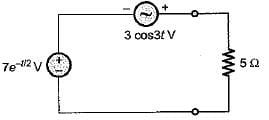Detailed Solution for Test: AC Power Analysis - 1 - Question 3

At t = 0, the given circuit is as shown below.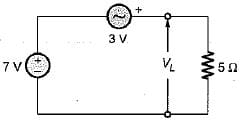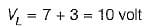∴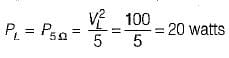Test: AC Power Analysis - 1 - Question 4

For a sinusoidal waveform, the ratio of average to rms value is

Detailed Solution for Test: AC Power Analysis - 1 - Question 4

For a sinusoidal waveform,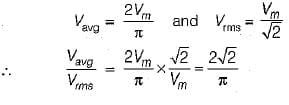Test: AC Power Analysis - 1 - Question 5

An impedance of (3 + j 4) Ω is connected in parallel with a resistance of 10 Ω. The ratio of power loss in these parallel circuits is

Detailed Solution for Test: AC Power Analysis - 1 - Question 5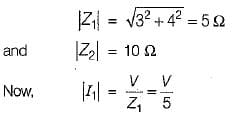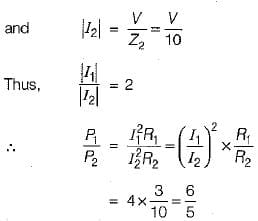Test: AC Power Analysis - 1 - Question 6

A 0.2 HP induction motor runs at an efficiency of 85%. If the operating power factor is 0.8 lag, the reactive power taken by the motor is

Detailed Solution for Test: AC Power Analysis - 1 - Question 6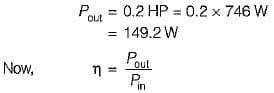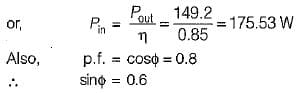∴ Reactive power taken by motor is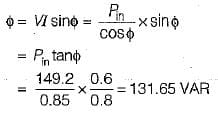Test: AC Power Analysis - 1 - Question 7

In an a.c. circuit, v = 100 sin (ωt + 30°) V, i = 5 sin(ωt- 30°) A. The apparant and reactive power in the circuit are respectively

Detailed Solution for Test: AC Power Analysis - 1 - Question 7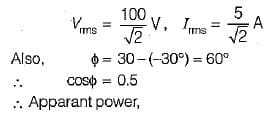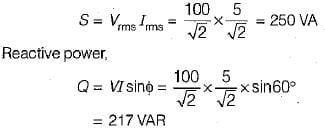Test: AC Power Analysis - 1 - Question 8

The inductive reactance in series with Z in the circuit shown below has a value of 25 Ω If the voltage drop a cross Z is 179 volts, the power dissipated in the circuit is 320 W.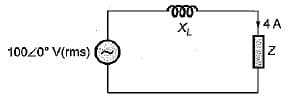The reactive part of Z is

Detailed Solution for Test: AC Power Analysis - 1 - Question 8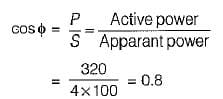Now, power dissipated,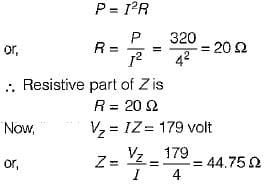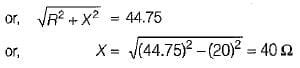Test: AC Power Analysis - 1 - Question 9

The values of current I1 and I2 in the circuit shown below are given by:

1A = (0.8 + j0.2) A and I2 = -(1 - j0.5) A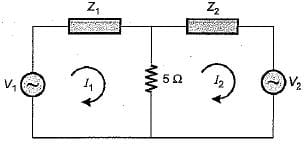The total power lost in the 5 Ω resistor is

Detailed Solution for Test: AC Power Analysis - 1 - Question 9

Power lost in the 5Ω resistor is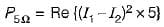(As current through 5 Ω resistor = I1 -I2)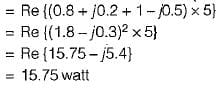Test: AC Power Analysis - 1 - Question 10

​The power factor of the circuit shown below is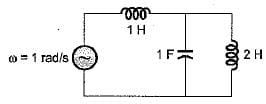Detailed Solution for Test: AC Power Analysis - 1 - Question 10

Given, ω = 1 rad/sec. So, the circuit can be redrawn as shown below.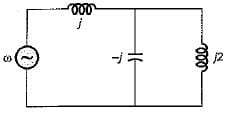Total impedance of the circuit is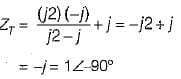∴ p.f. of the given circuit = cos90° = 0

## GATE Electrical Engineering (EE) 2023 Mock Test Series

22 docs|274 tests
Information about Test: AC Power Analysis - 1 Page
In this test you can find the Exam questions for Test: AC Power Analysis - 1 solved & explained in the simplest way possible. Besides giving Questions and answers for Test: AC Power Analysis - 1, EduRev gives you an ample number of Online tests for practice

## GATE Electrical Engineering (EE) 2023 Mock Test Series

22 docs|274 tests(Scan QR code)